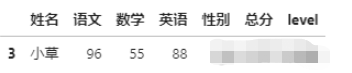# pandas调用函数怎么用

### 0. 数据预览

```import pandas as pd
df```
 姓名 语文 数学 英语 性别 总分 0 才哥 91 95 92 1 1 小明 82 93 91 1 2 小华 82 87 94 1 3 小草 96 55 88 0 4 小红 51 41 70 0 5 小花 58 59 40 0 6 小龙 70 55 59 1 7 杰克 53 44 42 1 8 韩梅梅 45 51 67 0

### 1. apply

`apply`可以对`DataFrame`类型数据按照列或行进行函数处理，默认情况下是按照（单独对`Series`亦可）。

```def getSex(s):
if s==1:
return '男'
elif s==0:
return '女'```

`df['性别'].apply(getSex)`

0 男
1 男
2 男
3 女
4 女
5 女
6 男
7 男
8 女
Name: 性别, dtype: object

`df['性别'].apply( lambda s: '男' if s==1 else '女' )`

0 男
1 男
2 男
3 女
4 女
5 女
6 男
7 男
8 女
Name: 性别, dtype: object

```# 多列条件组合
df['level'] = df.apply(lambda df: '高分' if df['总分']>=200 and df['数学']>=90 else '其他', axis=1)
df``````# python内置的函数
df[['语文','数学','英语','总分']].apply(max)```

dtype: int64

```# numpy自带的函数
import numpy as np
df[['语文','数学','英语','总分']].apply(np.mean)```

dtype: float64

### 2. applymap

`applymap`则是对每个元素的函数处理，变量是每个元素值。

`df[['语文','数学','英语']].applymap(lambda x:'高分' if x>=90 else '其他')`
 语文 数学 英语 0 高分 高分 1 其他 高分 2 其他 其他 3 高分 其他 4 其他 其他 5 其他 其他 6 其他 其他 7 其他 其他 8 其他 其他

### 3. map

`map`则是根据输入对应关系映射值返回最终数据，作用于`某一列`。传入的值可以是字典，键值为原始值，值为需要替换的值。也可以传入一个函数或者字符格式化表达式等等。

`df['性别'].map({1:'男', 0:'女'})`

0 男
1 男
2 男
3 女
4 女
5 女
6 男
7 男
8 女
Name: 性别, dtype: object

`df['总分'].map('总分：{}分'.format)`

0 总分：278分
1 总分：266分
2 总分：263分
3 总分：239分
4 总分：162分
5 总分：157分
6 总分：184分
7 总分：139分
8 总分：163分
Name: 总分, dtype: object

### 4. agg

`agg`一般用于聚合，在分组或透视操作中常见到，用法是和`apply`比较接近。

`df[['语文','数学','英语','总分']].agg(['max','min','mean'])````# 语文最高分、数学最低分和英文最高最低分
df.agg({'语文':['max'],'数学':'min','英语':['max','min']})```### 5. pipe

pipe又称管道方法，可以将我们的处理分析过程标准化、流程化。它在调用函数的时候可以带被调用函数的其他参数，这样就方便自定义函数的功能扩展了。

```# 定义一个函数，总分大于等于n，性别为sex的同学数据（sex为2表示不分性别）
def total(df, n, sex):
dfT = df.copy()
if sex == 2:
return dfT[(dfT['总分']>=n)]
else:
return dfT[(dfT['总分']>=n) & (dfT['性别']==sex)]```

`df.pipe(total,200,2)``df.pipe(total,150,1)``df.pipe(total,200,0)`